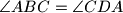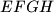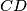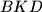### MEMO 2012 ekipno problem 6

Kvaliteta:
Avg: 3.0
Težina:
Avg: 6.0
Let$ABCD$ be a convex quadrilateral with no pair of parallel sides, such that$\angle ABC = \angle CDA$. Assume that the intersections of the pairs of neighbouring angle bisectors of$ABCD$ form a convex quadrilateral$EFGH$. Let$K$ be the intersection of the diagonals of$EFGH$. Prove that the lines$AB$ and$CD$ intersect on the circumcircle of the triangle$BKD$.
Source: Srednjoeuropska matematička olimpijada 2012, ekipno natjecanje, problem 6